# Conic Sections

### From DT Online

##### Description

A Cone can be described as the Locus of all lines joining points on the circumference of a ‘Base Circle’ to a point, or ‘Apex’, above it. If the Apex lies perpendicularly above the centre of the base circle it is known as a ‘right cone’ and if not, it is an ‘oblique cone’.

A right circular cone is a Solid of Revolution and can be produced by rotating a right angle triangle around one of the sides opposite its hypotenuse. The hypotenuse generates the surface of a cone as it sweeps round the central ‘Axis’. Any line joining the Apex to the Base Circle is known as a ‘Generator’. Generators can continue through the Apex to produce a second cone opposite to the first, creating a ‘Double Cone,’ and each Cone could extend beyond he Base Circles to infinity.

A right circular cone can be sliced across in various ways to produce a number of Conic Sections. These are curves with particular mathematical properties and which are used in engineering design - e.g. cam profiles and arch bridge design.

##### Constructions
 Ellipse The curve produced when a secton is taken across a right circular cone at an angle to the base circle, and intersecting the generators on both sides. Ellipses are a closed curve with a Major Axis and a Minor Axis. An Ellipse is also the result of taking a section across a Cylinder at at angle to its base. Ellipses describe the orbits of planets and the curve has been used to design Arch Bridges. Ellipses can be drawn as Loci with an Eccentricity of less than 1 (i.e. the Locus of all points where the ratio of distances from its Focus and Directrix are a constant which is less than 1)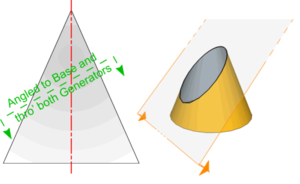Parabola The curve produced when a secton is taken through a right circular cone parallel to the generator. A Parabola describes the path taken by a object thrown into the air and the curve has been used to design Arch Bridges. Parabolas can be drawn as Loci with an Eccentricity equal to 1 (i.e. the Locus of all points where the ratio of distances from its Focus and Directrix are a constant which is equal to 1)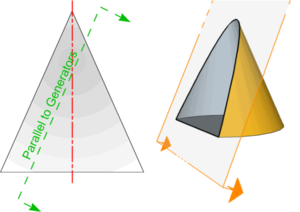Hyperbola The curve produced when a secton is taken which cuts both parts of a Double Cone but does not pass through the Apex (e.g. parallel to Axis as shown) - note that with a Double Cone, two Hyperbolae are created. Hyperbolae describe the paths taken by non-orbiting comets or the shadow cast on a sun dial for example. An Hyperbola can be drawn as a Locus with an Eccentricity greater than 1 (i.e. the Locus of all points where the ratio of distances from its Focus and Directrix are a constant which is greater than 1)Circle The curve produced when a secton is taken across a right circular cone parallel to the base circle. The circle can be considered as a fourth type of conic section (as it was by Apollonius) or as a special case of ellipse. The part of a cone (or Pyramid) lying between two parallel planes such as this is called the Frustrum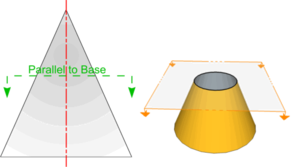Triangle Included for completeness, triangles are created when a section is taken through the Apex and across the Base Cicle diameter. Either an Isosceles or Equilaterial Triangle is revealed when a Right Cone is halved in this way. Other triangles would result from similar sectioning of an Oblique Cone.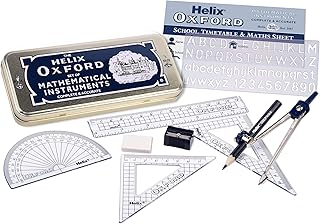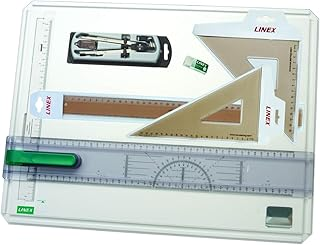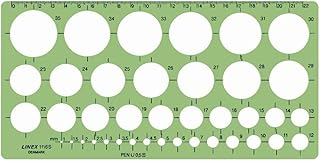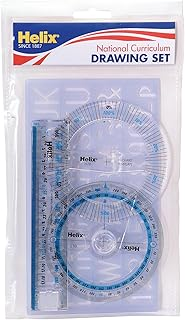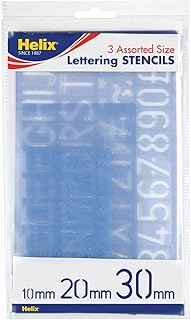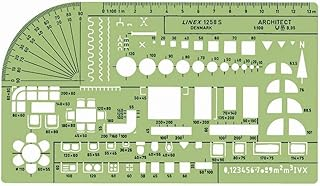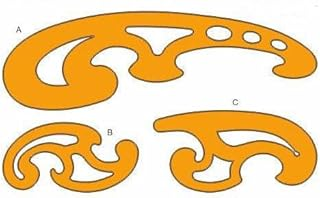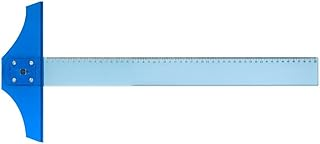Helix Oxford Maths SetLinex Student Saver BundleHelix Precision Plus Drawing SetPrimary Shape Template SetLinex Circle TemplateHelix National Curriculum Drawing SetHelix 3 Piece Stencil SetLinex Universal Architects TemplateFrench Curve Transparent TemplateT-Square